# CBSEtips.in

## Thursday, 25 February 2021

### CBSE Class 7 Maths - MCQ and Online Tests - Unit 15 - Visualising Solid Shapes

#### CBSE Class 7 Maths – MCQ and Online Tests – Unit 15 – Visualising Solid Shapes

Every year CBSE students attend Annual Assessment exams for 6,7,8,9,11th standards. These exams are very competitive to all the students. So our website provides online tests for all the 6,7,8,9,11th standards’ subjects. These tests are also very effective and useful for those who preparing for any competitive exams like Olympiad etc. It can boost their preparation level and confidence level by attempting these chapter wise online tests.

These online tests are based on latest CBSE syllabus. While attempting these, our students can identify their weak lessons and continuously practice those lessons for attaining high marks. It also helps to revise the NCERT textbooks thoroughly

#### CBSE Class 7 Maths – MCQ and Online Tests – Unit 15 – Visualising Solid Shapes

Question 1.
The number of edges of the solid shape is(a) 1
(b) 2
(c) 3
(d) 6

Question 2.
What cross-section do you get when you give a vertical cut to a brick?
(a) Square
(b) Rectangle
(c) Triangle
(d) Circle

Question 3.
The number of vertices of the solid shape is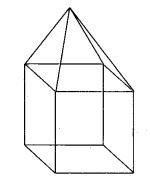(a) 9
(b) 4
(c) 6
(d) 8

Question 4.
The name of the solid shape is(a) cylinder
(b) cone
(c) sphere
(d) cube

Question 5.
The name of the solid shape is(a) cube
(b) cylinder
(c) cone
(d) sphere

Question 6.
The name of the solid shape is(a) cylinder
(b) cone
(c) cuboid
(d) sphere

Question 7.
The name of the solid shape is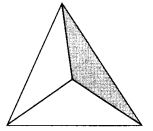(a) cylinder
(b) cone
(c) sphere
(d) pyramid

Question 8.
The name of the solid shape is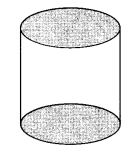(a) cone
(b) cylinder
(c) sphere
(d) cube

Question 9.
The name of the solid shape is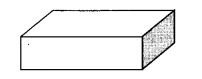(a) cuboid
(b) cube
(c) pyramid
(d) cone

Question 10.
The number of faces of a cube is
(a) 8
(b) 12
(c) 6
(d) 3

Question 11.
The number of vertices of the solid shape is(a) 1
(b) 2
(c) 3
(d) 4

Question 12.
The number of faces of the solid shape is(a) 1
(b) 2
(c) 3
(d) 4

Question 13.
What cross-section do you get when you give a horizontal cut to a brick?
(a) Triangle
(b) Circle
(c) Square
(d) Rectangle

Question 14.
What cross-section do you get when you give a vertical cut to a round apple?
(a) Circle
(b) Triangle
(c) Square
(d) Rectangle

Question 15.
The number of faces of the solid shape is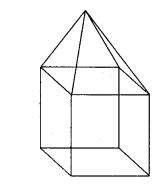(a) 4
(b) 6
(c) 9
(d) 8

Question 16.
The number of edges of the solid shape is(a) 16
(b) 9
(c) 6
(d) 4

Question 17.
Two cubes of edge length 2 cm are placed side by side. The length of the resulting cuboid is
(a) 2 cm
(b) 4 cm
(c) 1 cm
(d) none of these

Answer: (b) 4 cm

Question 18.
What cross-section do you get when you give a horizontal cut to a die?
(a) Square
(b) Rectangle
(c) Triangle
(d) Circle

Question 19.
The number of vertices of a cube is
(a) 8
(b) 12
(c) 6
(d) 3

Question 20.
The number of edges of a cube is
(a) 8
(b) 12
(c) 6
(d) 3